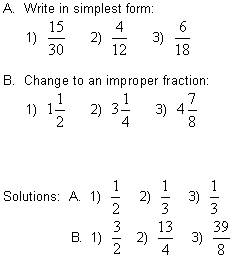# Equivalent Fractions Math Review

math tutorials > equivalent fractions

## Different ways of representing the same fraction are called equivalent fractions.

For example...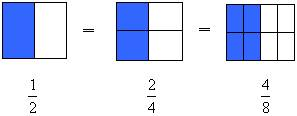Equivalent fractions can be formed by multiplying the numerator and denominator by the same number.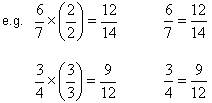## Simplifying Fractions

To simplify a fraction means to write it with it's smallest possible denominator.  This is also called cancelling.  Here, you divide the numerator and denominator by the same number.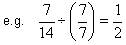## Improper Fractions

An improper fraction has the numerator larger than the denominator.  To change an improper fraction to a mixed number divide the numerator by the denominator.  The remainder is written as a fraction with the same denominator as the original improper fraction.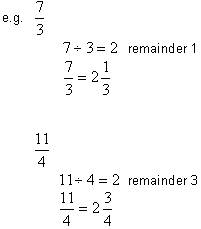## Mixed Numbers

To change from a mixed number to an improper fraction multiply the whole number by the denominator and add the result to the numerator.  This gives the numerator of the improper fraction.  The denominator remains the same.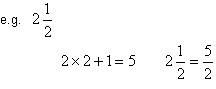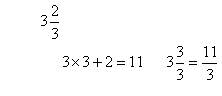## Equivalent fractions problems for you to try.